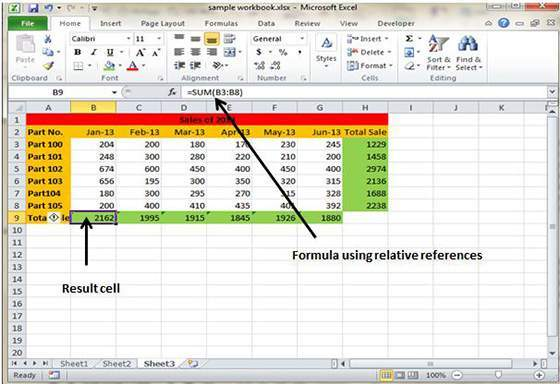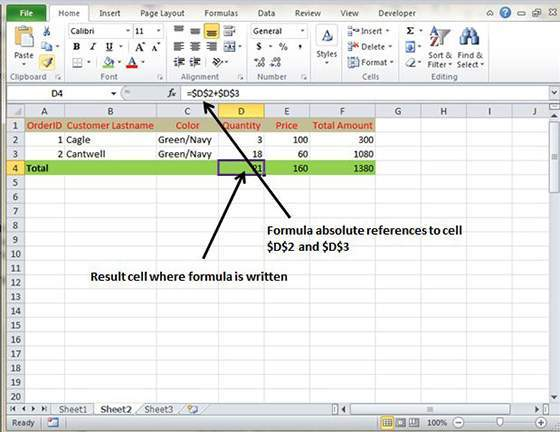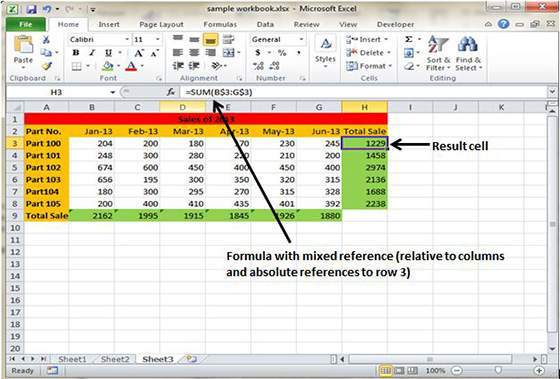# How To Use Formula Reference In The MS Excel 2010

Cell References in Formulas

Most of the formulas created by the user in MS Excel 2010 include the references to the cells or ranges. These references allow the formulas to work in the dynamic fashion.

It can be understood by this simple example. If your formula refers to the cell B2 and the value contained is changed by you then the result of the formula will also change automatically to show the new result.

In case you had not used the references in the formula then it has to be edited manually in order to the change the valued used in the formulas.

Most of the users make use of the cell reference in the formula as it happens to be dynamic and efficient in nature. There are three types of the references which can be used in the Ms Excel 2010 namely relative, absolute and mixed references.

Relative Cell Reference

Whenever you copy the formula to a new cell then the rows and columns references changes automatically. By default Excel is set at creating relative cell address in the formulas which allows easier use and implementation of same formulas throughout the worksheet.Absolute Cell References

When users try to copy the formula which contains actual cell addresses, then the row and column references doesn’t change and it results in returning of an error. It should be noted that an absolute reference uses two dollar signs in its address; one dollar sign for the column letter and one for the row number like this \$A\$5.Mixed Cell References

Either row or column reference is relative or the other happens to be absolute, then it is called mixed cell reference. In this, only one part of the address can be set at absolute like \$A or A\$5.